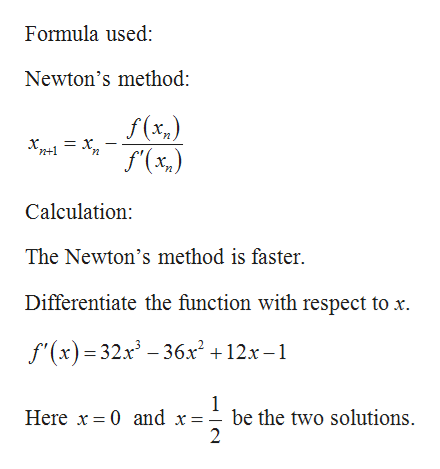# Consider solving the equation 8x4 - 12x3 + 6x2 -x =0with either Bisection Method or Newtons Method. For one of the solution x = (1/2). Decide which method will converge faster. How about the solution x = 0?

Question
36 views

Consider solving the equation 8x- 12x+ 6x-x =0

with either Bisection Method or Newtons Method. For one of the solution x = (1/2). Decide which method will converge faster. How about the solution x = 0?

check_circle

Step 1

Consider the equation f (x) = 8x4 – 12x3 + 6x2 – x = 0.

Find the method which converge...help_outlineImage TranscriptioncloseFormula used: Newton's method: f (x,) = Xn n+1 f'(x,) Calculation: The Newton's method is faster. Differentiate the function with respect to x. f'(x) = 32x – 36x² +12x – 1 Here x = 0 and x=- be the two solutions. || fullscreen

### Want to see the full answer?

See Solution

#### Want to see this answer and more?

Solutions are written by subject experts who are available 24/7. Questions are typically answered within 1 hour.*

See Solution
*Response times may vary by subject and question.
Tagged in

### Math